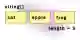# C# Array Length Property, Get Size of Array

Use the Length property on an array to get the size or element count of an array.

Length, array. An array has a length—this is its size (its element count). We access the Length property. An int of 0 or greater is returned—no iteration is done (a cache is used).ArrayLength notes. We see what happens when you get the Length of a one-dimensional array, an empty array, and a null array reference.

Initial Length example. Here we access Length on several instances of arrays. We see related properties, such as LongLength and the GetLength method.
Here: The program uses the Length property to get the length of a new array. Length has no parentheses, as it is a property.
Property
Empty: We get the length of an array with no elements. The Length property returns the value 0. This does not raise an exception.
Info: LongLength is the same as Length except it is returned as a long type. It can support bigger arrays too.
GetLength: This returns the length of an array at a dimension. We get dimension zero of a one-dimensional array.C# program that uses array Length property using System; class Program { static void Main() { // Basic array length example. string[] arrayA = new string[] { "cat", "apple", "frog" }; int lengthA = arrayA.Length; Console.WriteLine("LENGTH: " + lengthA); // Long array length example. long longLength = arrayA.LongLength; Console.WriteLine("LONGLENGTH: " + longLength); // Zero length array example. int[] zero = new int; int lengthZero = zero.Length; Console.WriteLine("LENGTH: " + lengthZero); // GetLength 0 example. int lengthE = arrayA.GetLength(0); Console.WriteLine("GETLENGTH: " + lengthE); } } Output LENGTH: 5 LONGLENGTH: 5 LENGTH: 0 GETLENGTH: 5

Two-dimensional length. Here we see a simple example of using GetLength on a two-dimensional array. GetLength receives a rank (0 or 1) and prints the result size.
Note: The Length property, when used on a 2D array, will return the total number of elements, not just a dimension.
Here: Length returns 5 * 10 elements, which is 50. Every element is counted in Length.
2D Array
C# program that uses GetLength, 2D array using System; class Program { static void Main() { // Two-dimensional GetLength example. int[,] two = new int[5, 10]; Console.WriteLine("GETLENGTH: " + two.GetLength(0)); // Writes 5 Console.WriteLine("GETLENGTH: " + two.GetLength(1)); // Writes 10 // Two-dimensional Length example. Console.WriteLine("LENGTH: " + two.Length); // Writes 50 } } Output GETLENGTH: 5 GETLENGTH: 10 LENGTH: 50

Null array length. This program will raise an exception when you run it. Because the array reference is null, you will get an exception.NullNullReferenceExceptionNull Array
Note: You receive "System.NullReferenceException: Object reference not set to an instance of an object."
C# program that causes null error using System; class Program { static void Main() { int[] test = null; Console.WriteLine(test.Length); } } Output Unhandled Exception: System.NullReferenceException: Object reference not set to an instance of an object. at Program.Main()...

Index error. GetLength can cause an exception when we access a dimension that is not part of the array. Here the array has one dimension, but we ask for the second dimension.
Text: The exception is "System.IndexOutOfRangeException: Index was outside the bounds of the array."
IndexOutOfRangeException
C# program that causes GetLength error using System; class Program { static void Main() { int[] test = new int; Console.WriteLine(test.GetLength(1)); } } Output Unhandled Exception: System.IndexOutOfRangeException: Index was outside the bounds of the array. at System.Array.GetLength(Int32 dimension) at Program.Main()...

LongLength. The array type offers also the LongLength property. This is only useful when you have an element count greater than the max value of an int.
Note: The max value of int is greater than 2 billion. If each array element is a byte, this comes to nearly 2 gigabytes of memory.
And: Depending on your system configuration, it may be impossible to allocate an array this large.

Discussion. Jagged arrays are essentially single-dimensional arrays of single-dimensional arrays. You can access their lengths the same as with one-dimensional arrays.Jagged Arrays

Count, LINQ. Count() is an extension method that acts on IEnumerable. You can use it to get the array length. It is many times slower and unnecessary for array types.Count

String arrays. Like all arrays, regardless of type, string arrays are of type Array and will have the Length property. Therefore, string arrays work the same as int arrays here.

Initialization. It does not matter if the array elements are initialized. You can get the length of any allocated array. Int array elements are initialized to 0.
Default: The default value of elements in an array can be determined with the default operator.
Default

Performance. In a tight loop where hoisting the Length check will not affect JIT, you can cache it in a variable for a performance boost.
Tip: It is a good idea to always test this. The JIT compiler sometimes generates slower instructions if you cache the length.
Also: The LongLength property, which returns a long number, has no performance advantage.

A summary. We saw lots of examples of different array instances and their Length properties. Further, we looked at the exceptions raised by Length and GetLength.

Home
© 2007-2020 Sam Allen. Send bug reports to info@dotnetperls.com.